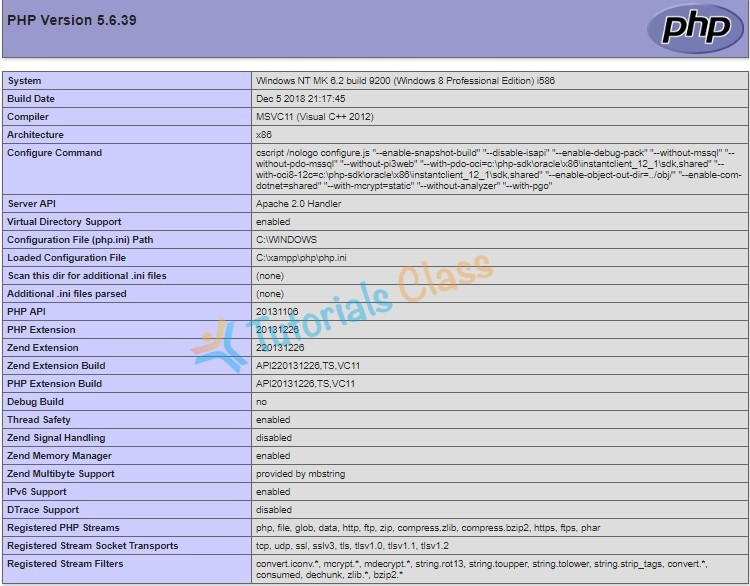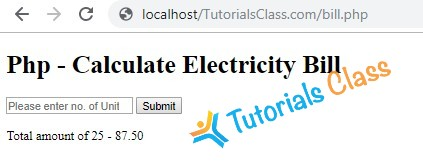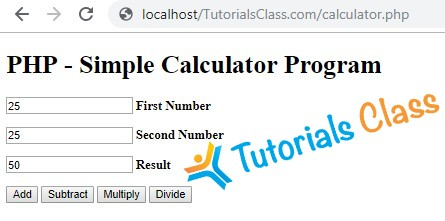# PHP All Exercises & Assignments

Practice your PHP skills using PHP Exercises & Assignments. Tutorials Class provides you exercises on PHP basics, variables, operators, loops, forms, and database.

Once you learn PHP, it is important to practice to understand PHP concepts. This will also help you with preparing for PHP Interview Questions.

Here, you will find a list of PHP programs, along with problem description and solution. These programs can also be used as assignments for PHP students.

## Write a program to count 5 to 15 using PHP loop

Description:
Write a Program to display count, from 5 to 15 using PHP loop as given below.

Rules & Hint

• You can use “for” or “while” loop
• You can use variable to initialize count
• You can use html tag for line break

View Solution/Program

``````<?php
\$count = 5;
while(\$count <= 15)
{
echo \$count;
echo "<br>" ;

\$count++;
}
?>``````
Tutorials Class - Output Window

5
6
7
8
9
10
11
12
13
14
15

## Write a program to print “Hello World” using echo

Description:
Write a program to print “Hello World” using echo only?

Conditions:

• You can not use any variable.

View Solution /Program

`````` <?php
echo "Hello World";
?>``````
Tutorials Class - Output Window

Hello World

## Write a program to print “Hello PHP” using variable

Description:
Write a program to print “Hello PHP” using php variable?

Conditions:

• You can not use text directly in echo but can use variable.

View Solution/Program

``````  <?php
\$message = "Hello PHP";
echo \$message;
?>``````
Tutorials Class - Output Window

Hello PHP

## Write a program to print a string using echo+variable.

Description:
Write a program to print “Welcome to the PHP World” using some part of the text in variable & some part directly in echo.

Conditions:

• You have to use a variable that contains string “PHP World”.

View Solution/Program

``````<?php
\$message = "Welcome to the PHP World";
echo \$message;
?>``````
Tutorials Class - Output Window

Welcome to the PHP World

## Write a program to print two variables in single echo

Description:
Write a program to print 2 php variables using single echo statement.

Conditions:

• First variable have text “Good Morning.”
• Second variable have text “Have a nice day!”
• Your output should be “Good morning. Have a nice day!”
• You are allowed to use only one echo statement in this program.

View Solution/Program

``````<?php
\$message_1 = "Good Morning.";
\$message_2 = "Have a nice day!";
echo \$message_1." ". \$message_2;
?>``````
Tutorials Class - Output Window

Good Morning. Have a nice day!

## Write a program to check student grade based on marks

Description:

Write a program to check student grade based on the marks using if-else statement.

Conditions:

• If marks are 60% or more, grade will be First Division.
• If marks between 45% to 59%, grade will be Second Division.
• If marks between 33% to 44%, grade will be Third Division.
• If marks are less than 33%, student will be Fail.

Click to View Solution/Program

``````<?php
\$marks = 40;

if (\$marks>=60)
{
}
else if(\$marks>=45)
{
}
else if(\$marks>=33)
{
}
else
{
}

?>``````
Tutorials Class - Output Window

Third Division

## Write a program to show day of the week using switch

Description:

Write a program to show day of the week (for example: Monday) based on numbers using switch/case statements.

Conditions:

• You can pass 1 to 7 number in switch
• Day 1 will be considered as Monday
• If number is not between 1 to 7, show invalid number in default

View Solution/Program

``````<?php
\$day = "5";

switch (\$day) {
case "1":
echo "It is Monday!";
break;
case "2":
echo "It is today!";
break;
case "3":
echo "It is Wednesday!";
break;
case "4":
echo "It is Thursday!";
break;
case "5":
echo "It is Friday!";
break;
case "6":
echo "It is Saturday!";
break;
case "7":
echo "It is Sunday!";
break;
default:
echo "Invalid number!";
}
?>``````
Tutorials Class - Output Window

It is Friday!

## Write a factorial program using for loop in php

Description:

Write a program to calculate factorial of a number using for loop in php.

``````<?php
\$num = 3;
\$factorial = 1;

for (\$x=\$num; \$x>=1; \$x--)
{
\$factorial = \$factorial * \$x;
}

echo "The factorial of \$num is \$factorial";
?>``````
Tutorials Class - Output Window

The factorial of 3 is 6

## Factorial program in PHP using recursive function

Exercise Description:
Write a PHP program to find factorial of a number using recursive function.

What is Recursive Function?

• A recursive function is a function that calls itself.

## Factorial program in PHP using recursive function

``````<?php
function factorial(\$number) {

if (\$number < 2) {
return 1;
} else {
return (\$number * factorial(\$number-1));
}
}

echo factorial(4);
?>``````
Tutorials Class - Output Window

24

## Write a program to create Chess board in PHP using for loop

Description:

Write a PHP program using nested for loop that creates a chess board.

Conditions:

• You can use html table having width=”400px” and take “30px” as cell height and width for check boxes.

View Solution/Program

``````<table width="400px" cellspacing="0px" cellpadding="0px" border="1px">
<?php
for(\$row=1;\$row<=8;\$row++)
{
echo "<tr>";
for(\$column=1;\$column<=8;\$column++)
{
\$total=\$row+\$column;
if(\$total%2==0)
{
echo "<td height=35px width=30px bgcolor=#FFFFFF></td>";
}
else
{
echo "<td height=35px width=30px bgcolor=#000000></td>";
}
}
echo "</tr>";
}
?>
</table>``````
Tutorials Class - Output Window## Write a Program to create given pattern with * using for loop

Description:
Write a Program to create following pattern using for loops:

```*
**
***
****
*****
******
*******
********
```

Rules

• You can use for or while loop
• You can use multiple (nested) loop to draw above pattern

View Solution/Program using two for loops

``````<?php
for(\$row=1;\$row<=8;\$row++)
{
for (\$star=1;\$star<=\$row;\$star++)
{
echo "*";
}
echo "<br>";
}
?>``````
Tutorials Class - Output Window

*
**
***
****
*****
******
*******
********

## Simple Tips for PHP Beginners

When a beginner start PHP programming, he often gets some syntax errors. Sometimes these are small errors but takes a lot of time to fix. This happens when we are not familiar with the basic syntax and do small mistakes in programs. These mistakes can be avoided if you practice more and taking care of small things.

I would like to say that it is never a good idea to become smart and start copying. This will save your time but you would not be able to understand PHP syntax. Rather, Type your program and get friendly with PHP code.

#### Follow Simple Tips for PHP Beginners to avoid errors in Programming

• Type your PHP program code manually. Do not just Copy Paste.
• Always create a new file for new code and keep backup of old files. This will make it easy to find old programs when needed.
• Keep your PHP files in organized folders rather than keeping all files in same folder.
• Use meaningful names for PHP files or folders. Some examples are: “`variable-test.php`“, “`loops.php`” etc. Do not just use “`abc.php`“, “`123.php`” or “`sample.php`
• Avoid space between file or folder names. Use hyphens` (-)` instead.
• Use lower case letters for file or folder names. This will help you make a consistent code

These points are not mandatory but they help you to make consistent and understandable code. Once you practice this for 20 to 30 PHP programs, you can go further with more standards.

The PHP Standard Recommendation (PSR) is a PHP specification published by the PHP Framework Interop Group.

## Experiment with Basic PHP Syntax Errors

When you start PHP Programming, you may face some programming errors. These errors stops your program execution. Sometimes you quickly find your solutions while sometimes it may take long time even if there is small mistake. It is important to get familiar with Basic PHP Syntax Errors

Basic Syntax errors occurs when we do not write PHP Code correctly. We cannot avoid all those errors but we can learn from them.

Here is a working PHP Code example to output a simple line.

``````<?php
echo "Hello World!";
?>``````

Output: Hello World!

It is better to experiment with PHP Basic code and see what errors happens.

• Remove semicolon from the end of second line and see what error occurs
• Remove double quote from “Hello World!” what error occurs
• Remove PHP ending statement “?>” error occurs
• Use “
• Try some space between “

Try above changes one at a time and see error. Observe What you did and what error happens.

Take care of the line number mentioned in error message. It will give you hint about the place where there is some mistake in the code.

Read Carefully Error message. Once you will understand the meaning of these basic error messages, you will be able to fix them later on easily.

Note: Most of the time error can be found in previous line instead of actual mentioned line. For example: If your program miss semicolon in line number 6, it will show error in line number 7.

## Using phpinfo() – Display PHP Configuration & Modules

`phpinfo()`  is a PHP built-in function used to display information about PHP’s configuration settings and modules.

When we install PHP, there are many additional modules also get installed. Most of them are enabled and some are disabled. These modules or extensions enhance PHP functionality. For example, the date-time extension provides some ready-made function related to date and time formatting. MySQL modules are integrated to deal with PHP Connections.

It is good to take a look on those extensions. Simply use

`phpinfo()` function as given below.

#### Example Using `phpinfo()` function

``````<?php
phpinfo();
?>``````
Tutorials Class - Output Window## Write a PHP program to add two numbers

#### Description:

Write a program to perform sum or addition of two numbers in PHP programming. You can use PHP Variables and Operators

## PHP Program to add two numbers:

``````<?php
\$first = 15;
\$second = 10;
\$sum = \$first + \$second;
echo "Result: ".\$sum;
?>``````
Tutorials Class - Output Window

Result: 25

## Write a program to calculate Electricity bill in PHP

Description:

You need to write a PHP program to calculate electricity bill using if-else conditions.

Conditions:

• For first 50 units – Rs. 3.50/unit
• For next 100 units – Rs. 4.00/unit
• For next 100 units – Rs. 5.20/unit
• For units above 250 – Rs. 6.50/unit
• You can use conditional statements.

View Solution/Program

``````<!DOCTYPE html>

<title>PHP - Calculate Electricity Bill</title>

<?php
\$result_str = \$result = '';
if (isset(\$_POST['unit-submit'])) {
\$units = \$_POST['units'];
if (!empty(\$units)) {
\$result = calculate_bill(\$units);
\$result_str = 'Total amount of ' . \$units . ' - ' . \$result;
}
}
/**
* To calculate electricity bill as per unit cost
*/
function calculate_bill(\$units) {
\$unit_cost_first = 3.50;
\$unit_cost_second = 4.00;
\$unit_cost_third = 5.20;
\$unit_cost_fourth = 6.50;

if(\$units <= 50) {
\$bill = \$units * \$unit_cost_first;
}
else if(\$units > 50 && \$units <= 100) {
\$temp = 50 * \$unit_cost_first;
\$remaining_units = \$units - 50;
\$bill = \$temp + (\$remaining_units * \$unit_cost_second);
}
else if(\$units > 100 && \$units <= 200) {
\$temp = (50 * 3.5) + (100 * \$unit_cost_second);
\$remaining_units = \$units - 150;
\$bill = \$temp + (\$remaining_units * \$unit_cost_third);
}
else {
\$temp = (50 * 3.5) + (100 * \$unit_cost_second) + (100 * \$unit_cost_third);
\$remaining_units = \$units - 250;
\$bill = \$temp + (\$remaining_units * \$unit_cost_fourth);
}
return number_format((float)\$bill, 2, '.', '');
}

?>

<body>
<div id="page-wrap">
<h1>Php - Calculate Electricity Bill</h1>

<form action="" method="post" id="quiz-form">
<input type="number" name="units" id="units" placeholder="Please enter no. of Units" />
<input type="submit" name="unit-submit" id="unit-submit" value="Submit" />
</form>

<div>
<?php echo '<br />' . \$result_str; ?>
</div>
</div>
</body>
</html>``````
Tutorials Class - Output Window## Write a simple calculator program in PHP using switch case

Description:

You need to write a simple calculator program in PHP using switch case.

Operations:

• Subtraction
• Multiplication
• Division

View Solution/Program

``````<!DOCTYPE html>

<title>Simple Calculator Program in PHP - Tutorials Class</title>

<?php
\$first_num = \$_POST['first_num'];
\$second_num = \$_POST['second_num'];
\$operator = \$_POST['operator'];
\$result = '';
if (is_numeric(\$first_num) && is_numeric(\$second_num)) {
switch (\$operator) {
\$result = \$first_num + \$second_num;
break;
case "Subtract":
\$result = \$first_num - \$second_num;
break;
case "Multiply":
\$result = \$first_num * \$second_num;
break;
case "Divide":
\$result = \$first_num / \$second_num;
}
}

?>

<body>
<div id="page-wrap">
<h1>PHP - Simple Calculator Program</h1>
<form action="" method="post" id="quiz-form">
<p>
<input type="number" name="first_num" id="first_num" required="required" value="<?php echo \$first_num; ?>" /> <b>First Number</b>
</p>
<p>
<input type="number" name="second_num" id="second_num" required="required" value="<?php echo \$second_num; ?>" /> <b>Second Number</b>
</p>
<p>
</p>
<input type="submit" name="operator" value="Subtract" />
<input type="submit" name="operator" value="Multiply" />
<input type="submit" name="operator" value="Divide" />
</form>
</div>
</body>
</html>``````
Tutorials Class - Output Window## Remove specific element by value from an array in PHP?

Description:

You need to write a program in PHP to remove specific element by value from an array using PHP program.

Instructions:

• Take an array with list of month names.
• Take a variable with the name of value to be deleted.
• You can use PHP `array `functions or `foreach `loop.

### Solution 1: Using `array_search()`

With the help of `array_search()` function, we can remove specific elements from an array.

``````<?php
\$delete_item = 'march';
// take a list of months in an array
\$months = array('jan', 'feb', 'march', 'april', 'may');
if ((\$key = array_search(\$delete_item, \$months)) !== false) {
unset(\$months[\$key]);
}

// print array to see latest values
var_dump(\$months);
?>``````
Tutorials Class - Output Window

array(4) { => string(3) “jan” => string(3) “feb” => string(5) “april” => string(3) “may” }

### Solution 2: Using `foreach()`

By using `foreach()` loop, we can also remove specific elements from an array.

``````<?php
\$delete_item = 'april';
// take a list of months in an array
\$months = array('jan', 'feb', 'march', 'april', 'may'); // for april, the key is 4
foreach (array_keys(\$months, \$delete_item) as \$key) {
unset(\$months[\$key]);
}

// print array to see latest values
var_dump(\$months);
?>``````
Tutorials Class - Output Window

array(4) { => string(3) “jan” => string(3) “feb” => string(5) “april” => string(3) “may” }

### Solution 3: Using `array_diff()`

With the help of `array_diff()` function, we also can remove specific elements from an array.

``````<?php
\$delete_item = 'april';
// take a list of months in an array
\$months= array('jan', 'feb', 'march', 'april', 'may');
\$final_months= array_diff(\$months, array(\$delete_item));

// print array to see latest values
var_dump(\$final_months);
?>``````
Tutorials Class - Output Window

array(4) { => string(3) “jan” => string(3) “feb” => string(5) “march” => string(3) “may” }

## Write a PHP program to check if a person is eligible to vote

#### Description:

Write a PHP program to check if a person is eligible to vote or not.

##### Click to View Solution/Program.
``````<?php
function check_vote() //function has been declared
{
\$name = "Rakesh";
\$age = 19;
if (\$age >= 18) {
echo \$name . ", you Are Eligible For Vote";
} else {
echo \$name . ", you are not eligible for vote. ";
}
}
check_vote(); //function has been called

?>``````
Tutorials Class - Output Window

You Are Eligible For Vote

## Write a PHP program to calculate area of rectangle

#### Description:

Write a PHP program to calculate area of rectangle by using PHP Function.

#### Condition

• You must use a PHP Function.
• There should be two arguments i.e. length & width.
##### View Solution/Program.
``````<?php
function rect_area(\$length = 2, \$width = 4) //function has declared
{
\$area = \$length * \$width;
echo "Area Of Rectangle with length " . \$length . " & width " . \$width . " is " . \$area ;
}
rect_area(); // function has been called.

?>``````
Tutorials Class - Output Window

Area Of Rectangle with length 2 & width 4 is 8 .